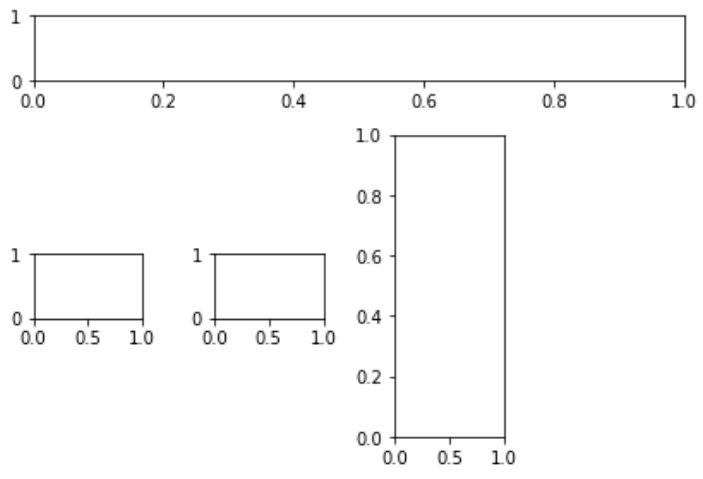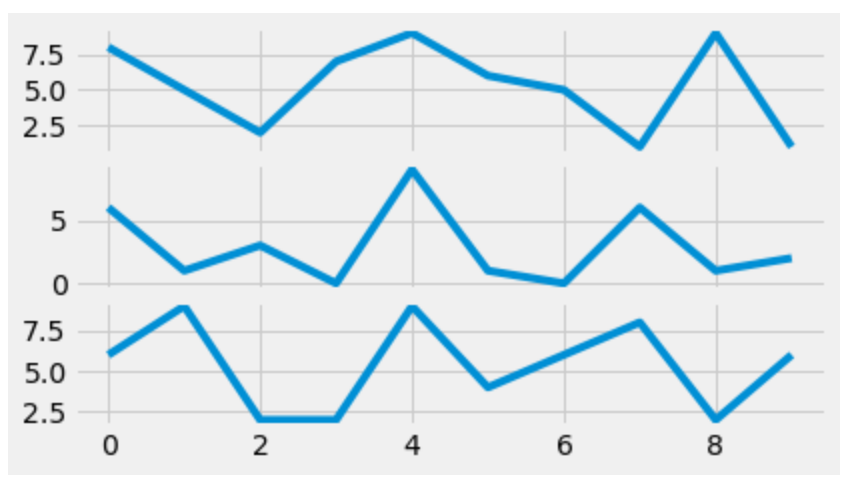Related Articles

# Matplotlib.pyplot.subplot2grid() in python

• Last Updated : 19 Apr, 2020

Matplotlib is an amazing visualization library in Python for 2D plots of arrays. Matplotlib is a multi-platform data visualization library built on NumPy arrays and designed to work with the broader SciPy stack.

## Matplotlib.pyplot.subplot2grid()

The Matplotlib.pyplot.subplot2grid() function give additional flexibility in creating axes object at a specified location inside a grid. It also helps in spanning the axes object across multiple rows or columns. In simpler words, this function is used to create multiple charts within the same figure. It is a sub-figure layout manager.

Syntax : Plt.subplot2grid(shape, location, rowspan, colspan)

Parameters :

• shape: As the name suggests it is used to define the shape of the grid to be plotted within the graph. It is a required argument and is generally passed in as a list or tuple of two numbers which are responsible for the layout of the grid with the first number being the number of rows and the second number as the number of columns.
• location (loc): This is the second mandatory argument that this function takes. Similar to the shape argument it is also a required argument and is generally passed in as a list or tuple of two numbers. It is used for specifying the row and column number to place the sub-plot. It is also important to note that the indexes start from 0. So (0, 0) would be the cell in the first row and the first column of the grid.
• rowspan: Once the grid layout is set and the starting index is decided using location(loc) one can expand the selection to take up more rows with this argument. This is an optional parameter and has a default value of 1.
• colspan: Similar to rowspan it is used to expand the selection to take up more columns. It is also an optional parameter with default value of 1.

Example 1:

 `import` `matplotlib.pyplot as plt`` ` ` ` `fig ``=` `plt.figure()`` ` `axes1 ``=` `plt.subplot2grid((``4``, ``4``), (``0``, ``0``),``                         ``colspan ``=` `4``)`` ` `axes2 ``=` `plt.subplot2grid((``4``, ``4``), (``1``, ``0``),``                         ``colspan ``=` `3``)`` ` `axes3 ``=` `plt.subplot2grid((``4``, ``4``), (``1``, ``2``), ``                         ``rowspan ``=` `3``)`` ` `axes4 ``=` `plt.subplot2grid((``4``, ``4``), (``2``, ``0``))``axes5 ``=` `plt.subplot2grid((``4``, ``4``), (``2``, ``1``))`` ` `fig.tight_layout()`

Output :Example 2:

 `import` `random``import` `matplotlib.pyplot as plt``from` `matplotlib ``import` `style`` ` ` ` `style.use(``'fivethirtyeight'``)`` ` `fig ``=` `plt.figure()`` ` ` ` `# helper function to plot the lines``def` `helper():``     ` `    ``xs ``=` `[]``    ``ys ``=` `[]`` ` `    ``for` `i ``in` `range``(``10``):``        ``x ``=` `i``        ``y ``=` `random.randrange(``10``)`` ` `        ``xs.append(x)``        ``ys.append(y)``    ``return` `xs, ys`` ` `axes1 ``=` `plt.subplot2grid ((``7``, ``1``), (``0``, ``0``),``                          ``rowspan ``=` `2``, ``                          ``colspan ``=` `1``)`` ` `axes2 ``=` `plt.subplot2grid ((``7``, ``1``), (``2``, ``0``),``                          ``rowspan ``=` `2``,``                          ``colspan ``=` `1``)`` ` `axes3 ``=` `plt.subplot2grid ((``7``, ``1``), (``4``, ``0``), ``                          ``rowspan ``=` `2``, ``                          ``colspan ``=` `1``)`` ` `x, y ``=` `helper()``axes1.plot(x, y)`` ` `x, y ``=` `helper()``axes2.plot(x, y)`` ` `x, y ``=` `helper()``axes3.plot(x, y)`

Output:Attention geek! Strengthen your foundations with the Python Programming Foundation Course and learn the basics.

To begin with, your interview preparations Enhance your Data Structures concepts with the Python DS Course. And to begin with your Machine Learning Journey, join the Machine Learning – Basic Level Course

My Personal Notes arrow_drop_up